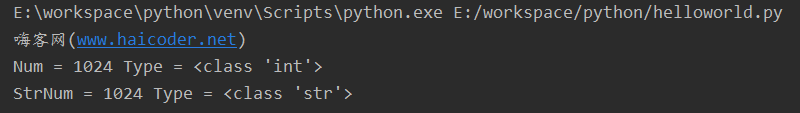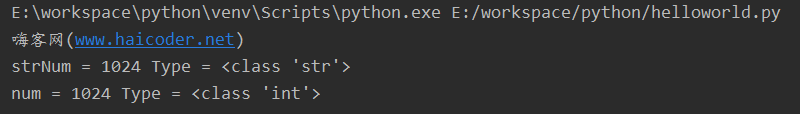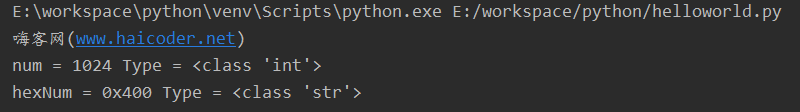• Python类型转换函数函数格式使用示例描述int(x [,base])int(“8”)可以转换的包括String类型和其他数字类型，但是会丢失精度float(x)float(1)或者float(“1”)可以转换String和其他数字类型，不足的位数用0补齐，...
Python类型转换教程虽然对 Python 内置的数据类型进行转换时，可以使用内置函数。Python类型转换函数函数格式使用示例描述int(x [,base])int(“8”)可以转换的包括String类型和其他数字类型，但是会丢失精度float(x)float(1)或者float(“1”)可以转换String和其他数字类型，不足的位数用0补齐，例如1会变成1.0complex(real ,imag)complex(“1”)或者complex(1,2)第一个参数可以是String或者数字，第二个参数只能为数字类型，第二个参数没有时默认为0str(x)str(1)将数字转化为Stringrepr(x)repr(Object)返回一个对象的String格式eval(str)eval(“12+23”)执行一个字符串表达式，返回计算的结果,如例子中返回35tuple(seq)tuple((1,2,3,4))参数可以是元组、列表或者字典，wie字典时，返回字典的key组成的集合list(s)list((1,2,3,4))将序列转变成一个列表，参数可为元组、字典、列表，为字典时，返回字典的key组成的集合set(s)set([‘b’, ‘r’, ‘u’, ‘o’, ‘n’])或者set(“asdfg”)将一个可以迭代对象转变为可变集合，并且去重复，返回结果可以用来计算差集x - y、并集x | y、交集x & yfrozenset(s)frozenset([0, 1, 2, 3, 4, 5, 6, 7, 8, 9])将一个可迭代对象转变成不可变集合，参数为元组、字典、列表等，chr(x)chr(0x30)chr()用一个范围在 range(256)内的(就是0～255)整数作参数，返回一个对应的字符。返回值是当前整数对应的ascii字符。ord(x)ord(‘a’)返回对应的 ASCII 数值，或者 Unicode 数值hex(x)hex(12)把一个整数转换为十六进制字符串oct(x)oct(12)把一个整数转换为八进制字符串案例数字转字符串使用 str() 函数，将print("嗨客网(www.haicoder.net)")# 使用 str 函数，将整数转成字符串num = 1024strNum = str(num)print('Num =', num, 'Type =', type(num))print('StrNum =', strNum, 'Type =', type(strNum))程序运行后，控制台输出如下：首先，我们定义了一个整型类型的变量，接着，我们使用 str() 函数，将整型变量转换成字符串类型。我们使用 print() 函数，输出变量 num 的值，以及变量 num 的类型，num 的值为 1024，类型为 int。最后，使用 print() 函数，输出变量 strNum 的值，以及变量 strNum 的类型，变量 strNum 的值为 1024，类型为 str，即整型变量 num 被我们使用 str 函数转换成了字符串类型。字符串转数字使用 int() 函数，将字符串转数字print("嗨客网(www.haicoder.net)")# 使用 int 函数，将字符串转成数字strNum = "1024"num = int(strNum)print('strNum =', strNum, 'Type =', type(strNum))print('num =', num, 'Type =', type(num))程序运行后，控制台输出如下：首先，我们定义了一个字符串类型的变量，接着，我们使用 int() 函数，将字符串变量转换成数字类型。我们使用 print() 函数，输出变量 strNum 的值，以及变量 strNum 的类型，变量 strNum 的值为 1024，类型为 str。最后，使用 print() 函数，输出变量 num 的值，以及变量 num 的类型，num 的值为 1024，类型为 int，即字符串变量 strNum 被我们使用 int 函数转换成了数字类型。十进制转十六进制使用 hex() 函数，将十进制转十六进制print("嗨客网(www.haicoder.net)")# 使用 hex 函数，将十进制转十六进制num = 1024hexNum = hex(num)print('num =', num, 'Type =', type(num))print('hexNum =', hexNum, 'Type =', type(hexNum))程序运行后，控制台输出如下：首先，我们定义了一个 int 类型的变量，接着，我们使用 hex() 函数，将 int 变量转换成十六进制的字符串类型。我们使用 print() 函数，输出变量 num 的值，以及变量 num 的类型，变量 num 的值为 1024，类型为 int。最后，使用 print() 函数，输出变量 hexNum 的值，以及变量 hexNum 的类型，hexNum 的值为 0x400，类型为 str，即 int 变量 num 被我们使用 hex 函数转换成了十六进制的字符串类型。Python类型转换总结对 Python 内置的数据类型进行转换时，可以使用内置函数。
展开全文• Python 强制类型转换 文章目录Python 强制类型转换几种强制类型转换实例1. 整数和浮点数之间的强制类型转换2. 列表、元组、集合之间的相互转换3. 整型、浮点数与字符串之间的相互转换 python 中的数据类型有以下几种...
Python 强制类型转换
文章目录Python 强制类型转换几种强制类型转换实例1. 整数和浮点数之间的强制类型转换2. 列表、元组、集合之间的相互转换3. 整型、浮点数与字符串之间的相互转换
python 中的数据类型有以下几种
int		# 整型	1
float	# 浮点型	1.0
str		# 字符串型	'1'
tuple	# 元组	(1)
list	# 列表	
dict	# 字典	{'':'1'}
set		# 集合	{1}

既然存在几种不同类型的数据，python 也提供了几种称为强制类型转换的函数
int(x)             # 将x转换为一个整数
float(x)           # 将x转换到一个浮点数
str(x)             # 将对象 x 转换为字符串
tuple(s)           # 将序列 s 转换为一个元组
list(s)            # 将序列 s 转换为一个列表
set(s)             # 将序列 s 转换为一个集合

几种强制类型转换实例
1. 整数和浮点数之间的强制类型转换
a = 1
print("type of a: ",type(a))
print(a)
b = float(a)
print("type of b: ",type(b))
print(b)

# type of a:  <class 'int'>
# 1
# type of b:  <class 'float'>
# 1.0

2. 列表、元组、集合之间的相互转换
a = [1, 2, 3]
print("type of a: ",type(a))
print(a)
b = tuple(a)
print("type of b: ",type(b))
print(b)
c = set(a)
print("type of c: ",type(c))
print(c)
e = set(b)
print("type of e: ",type(e))
print(e)
print(c,e)
# d = dict(a)
# print("type of d: ",type(d))
# print(d)
# TypeError: cannot convert dictionary update sequence element #0 to a sequence

# type of a:  <class 'list'>
# [1, 2, 3]
# type of b:  <class 'tuple'>
# (1, 2, 3)
# type of c:  <class 'set'>
# {1, 2, 3}
# type of e:  <class 'set'>
# {1, 2, 3}
# {1, 2, 3} {1, 2, 3}

3. 整型、浮点数与字符串之间的相互转换
a = '12'
print("type of a: ",type(a))
print(a)
b = int(a)
print("type of b: ",type(b))
print(b)
c = float(a)
print("type of c: ",type(c))
print(c)
d = 123
print("type of d: ",type(d))
print(d)
e = str(d)
print("type of e: ",type(e))
print(e)

# type of a:  <class 'str'>
# 12
# type of b:  <class 'int'>
# 12
# type of c:  <class 'float'>
# 12.0
# type of d:  <class 'int'>
# 123
# type of e:  <class 'str'>
# 123



展开全文• #### 强制类型转换 Number (int float bool complex)var1= 13var2= 13.789var3=Truevar4= 5-7jvar5= "9988"var6= "abcd4567"#(1) int 强制转换成整型res = int(var2) #13res = int(var3) #False => 0 True => ...
#### 强制类型转换 Number (int float bool complex)var1= 13var2= 13.789var3=Truevar4= 5-7jvar5= "9988"var6= "abcd4567"#(1) int 强制转换成整型res = int(var2) #13res = int(var3) #False => 0 True => 1#res = int(var4) errorres = int(var5) #9988#res = int(var6) errorprint(res , type(res))#(2) float 强制转换成浮点型res = float(var1) #13.0res = float(var3) #True => 1.0 False => 0.0res = float(var5) #9988.0print(res , type(res))#(3) complex 强制转换成复数res = complex(var1) #13 + 0jres = complex(var2) #13.789 + 0jres = complex(var3) #False => 0j True => 1+0jres = complex(var5) #9988 + 0j#res = complex(var6) errorprint(res , type(res))#(4) bool 强制转换成布尔型 (***)"""bool类型为假的十种情况0 , 0.0 , False , 0j , '',[],(),set(),{},None"""res=bool(None)print(res , type(res))#None 是python的关键字,代表空的,什么也没有,一般用来做初始化操作a1 =Noneb1=None"""总结:int() float() bool() complex()默认创建一个该数据类型的值res = int()res = float()res = bool()res = complex()print(res)"""#### 自动类型转换 Number( int float bool complex)"""精度从低向高进行转换 : bool -> int -> float -> complex自动类型转换,默认从低精度向高精度进行转换(从低到高)"""#1.bool + intres = True + 100 #1 + 100print(res)#2.bool + floatres = True + 4.15 #1.0 + 4.15print(res)#3.bool + complexres = True + 3-6j #1+0j + 3 - 6jprint(res)#4.int + floatres = 5 + 3.48 #5.0 + 3.48print(res)#5.int + complexres = 10 + 4-3j #10 + 0j + 4 - 3jprint(res)#6.float + complexres = 5.68 + 5-100j #5.68+0j + 5 - 100jprint(res)#### 容器类型的强制转换 (str  list tuple set dict)#推荐大家使用变量命名的字符串作为字典的键;dictvar = {"lzh":"气质非凡","mcp":"高大威猛","lxw":"气宇轩昂"}intvar= 8888#(1) str (容器 / Number 都可以转换)"""强转成字符串,无非就是在原有的数据的基础上两边套上引号"""res=str(listvar)res=str(intvar)print(res , type(res))#repr 可以原型化输出字符串,不转义字符(显示出引号)print( repr(res) )#(2) list : 强制转换成列表"""如果是字符串,会把每一个字符单独的作为一个元素放到列表中如果是字典,只保留键,形成一套新的列表如果是其他的容器,只是单纯的在原有数据的基础上换上[]"""res=list(strvar)res=list(tuplevar)res=list(setvar)res=list(dictvar)print(res , type(res))#(3) tuple : 强制转换成元组"""如果是字符串,会把每一个字符单独的作为一个元素放到元组中如果是字典,只保留键,形成一套新的元组如果是其他的容器,只是单纯的在原有数据的基础上换上()"""res=tuple(strvar)res=tuple(listvar)res=tuple(setvar)res=tuple(dictvar)print(res , type(res))#(4) set : 强制转换成集合"""如果是字符串,会把每一个字符单独的作为一个元素放到集合中(无序,自动去重)如果是字典,只保留键,形成一套新的集合如果是其他的容器,只是单纯的在原有数据的基础上换上{}"""res=set(strvar)res=set(listvar)res=set(dictvar)print(res)#去掉列表中所有重复的数据lst = ['a','b','c','d','d','d',100,200]res=list( set(lst) )print(res)#### 二级容器 (list tuple set dict)#二级列表lst = [1,2,[3,4]]#二级元组tup = (5,6,7,(8,9))#二级集合setvar = {10,11,(12,13)}#二级字典dic ={"a":1,"b":{"c":3,"d":4}}#获取4这个元素res = dic["b"]print(res) #{'c': 3, 'd': 4}res2= res["d"]print(res2)#简写一步res = dic["b"]["d"]print(res)#五级容器container = [100,200,300,(1,2,3,4,{"a":1,"b":{"c":15,"d":[11,12,"bingo"]}})]#获取bingores1 = container[-1]print(res1) #(1, 2, 3, 4, {'a': 1, 'b': {'c': 15, 'd': [11, 12, 'bingo']}})res2= res1[-1]print(res2) #{'a': 1, 'b': {'c': 15, 'd': [11, 12, 'bingo']}}res3= res2["b"]print(res3) #{'c': 15, 'd': [11, 12, 'bingo']}res4= res3["d"]print(res4) #[11, 12, 'bingo']res5= res4[-1]print(res5)#简写一步res = container[-1][-1]["b"]["d"][-1]print(res) #bingo#等长的二级容器 : 里面的元素都是容器,并且容器里面的元素个数都相同lst = [(1,2,3) , [4,5,6]]lst= [(1,2) , [4,5]]#### dict 强制转换成字典"""强转成字典时 , 必须是等长的二级容器,里面的元素个数是2个"""#(1) 外层是列表或者元组或者集合,里面的容器是元组或者列表(推荐)lst = [("a",1),["b",2]]tup= (("c",3),["d",4],)setvar= {(1,2),(3,4),("f3",3),("f4",4)}res= dict(lst) #{'a': 1, 'b': 2}res = dict(tup) #{'c': 3, 'd': 4}#res = dict(setvar) # {1: 2, 'f3': 3, 3: 4, 'f4': 4}print(res)#(2) 如何里面是集合,语法上允许,但是有局限性(不推荐)lst = [{"a",333},{"zz",5}] #因为集合无序,不符合定义的本意,不推荐使用print(dict(lst))#(3) 如果使用字符串,语法上正确,但是有局限性(不推荐)lst = [("a",1),"b2"] #字符串长度只能2个#lst = [("a",1),"b23"] errorprint(  dict(lst)  )"""总结:str()  list()  tuple() dict() set()默认创建一个该数据类型的值"""res=set()print(res)#### 变量的缓存机制 (仅对python3.6版本负责)"""机制: 只有两个值相同,就只开辟一个空间python3.7python3.8"""#-->Number 部分#1.对于整型而言，-5~正无穷范围内的相同值 id一致var1 = 100var2= 100var1= -6var2= -6var1= var2 = -100 #相同的地址print(id(var1)   , id(var2))#2.对于浮点数而言，非负数范围内的相同值 id一致var1 = -5.76var2= -5.76print( id(var1) , id(var2) )#3.布尔值而言,值相同情况下，id一致var1 =Truevar2=Falseprint( id(var1) , id(var2) )#4.复数在 实数+虚数 这样的结构中永不相同(只有虚数的情况例外)var1 = 3+4jvar2= 3+4jvar1= 90jvar2= 90jprint( id(var1) , id(var2) )#-->容器类型部分#5.字符串 和 空元组 相同的情况下，地址相同var1 = "你"var2= "你"var1=()var2=()print( id(var1) , id(var2) )#6.列表，元组，字典，集合无论什么情况 id标识都不同 [空元组例外]var1 = (1,2,3)var2= (1,2,3)print( id(var1) , id(var2) )
展开全文• int(x [,base ]) 将x转换为一个整数long(x [,base ]) 将x转换为一个长整数float(x ) 将x转换到一个浮点数complex(real [,imag ]) 创建一个复数str(x ) 将对象 x 转换为字符串repr(x ) 将对象 x 转换为...
int(x [,base ])         将x转换为一个整数long(x [,base ])        将x转换为一个长整数float(x )               将x转换到一个浮点数complex(real [,imag ])  创建一个复数str(x )                 将对象 x 转换为字符串repr(x )                将对象 x 转换为表达式字符串eval(str )              用来计算在字符串中的有效Python表达式,并返回一个对象tuple(s )               将序列 s 转换为一个元组list(s )                将序列 s 转换为一个列表chr(x )                 将一个整数转换为一个字符unichr(x )              将一个整数转换为Unicode字符ord(x )                 将一个字符转换为它的整数值hex(x )                 将一个整数转换为一个十六进制字符串oct(x )                 将一个整数转换为一个八进制字符串做的DEMO：#类型转换#convert#convert to intprint('int()默认情况下为：', int())print('str字符型转换为int：', int('010'))print('float浮点型转换为int：', int(234.23))#十进制数10，对应的2进制，8进制，10进制，16进制分别是：1010,12,10,0xaprint('int(\'0xa\', 16) = ', int('0xa', 16))print('int(\'10\', 10) = ', int('10', 10))print('int(\'12\', 8) = ', int('12', 8))print('int(\'1010\', 2) = ', int('1010', 2))#convert to longprint('int浮点型转换为int：', int(23))#convert to floatprint('float()默认情况下为：', float())print('str字符型转换为float：', float('123.01'))print('int浮点型转换为float：', float(32))#covert to complexprint('创建一个复数(实部+虚部)：', complex(12, 43))print('创建一个复数(实部+虚部)：', complex(12))#convert to strprint('str()默认情况下为：', str())print('float字符型转换为str：', str(232.33))print('int浮点型转换为str：', str(32))lists = ['a', 'b', 'e', 'c', 'd', 'a']print('列表list转换为str:', ''.join(lists))#covert to liststrs = 'hongten'print('序列strs转换为list:', list(strs))#covert to tupleprint('列表list转换为tuple:', tuple(lists))#字符和整数之间的转换#char coverted to intprint('整数转换为字符chr:', chr(67))print('字符chr转换为整数:', ord('C'))print('整数转16进制数:', hex(12))print('整数转8进制数:', oct(12))运行结果：Python 3.3.2 (v3.3.2:d047928ae3f6, May 16 2013, 00:03:43) [MSC v.1600 32 bit (Intel)] on win32Type "copyright", "credits" or "license()" for more information.>>> ================================ RESTART ================================>>>int()默认情况下为： 0str字符型转换为int： 10float浮点型转换为int： 234int('0xa', 16) =  10int('10', 10) =  10int('12', 8) =  10int('1010', 2) =  10int浮点型转换为int： 23float()默认情况下为： 0.0str字符型转换为float： 123.01int浮点型转换为float： 32.0创建一个复数(实部+虚部)： (12+43j)创建一个复数(实部+虚部)： (12+0j)str()默认情况下为：float字符型转换为str： 232.33int浮点型转换为str： 32列表list转换为str: abecda序列strs转换为list: ['h', 'o', 'n', 'g', 't', 'e', 'n']列表list转换为tuple: ('a', 'b', 'e', 'c', 'd', 'a')整数转换为字符chr: C字符chr转换为整数: 67整数转16进制数: 0xc整数转8进制数: 0o14>>>
展开全文• Number类型的数据转换强制转换int可以转换的数据类型int 整型float 浮点型bool 布尔型str 字符串(整型)数据转换# 整型(整型转换是原封不动的)print(int(10))# 浮点型(浮点型转成整型按照退一法)print(int(10.999))...
• python2.4版本以后，如果int的值超出范围不会溢出，而是内部转换为long，在网上没有找到从long型强制转换int的代码，这里所说的int取值范围是和java里一致，即用四个字节表示。自己写了一个函数，勉强可以用，供...
• 强制类型转换： 根据程序需要，人为改变数据类型的方式称为强制数据类型转换(显式转换)。 1. int() 将其他类型转换为整型 基本语法如下： class int(x, base=10) 其中，x代表字符串或数字；base则是进制数，默认值为...
• ## python强制类型转换astype

万次阅读 多人点赞 2019-01-08 11:56:30
在进行将多个表的数据合并到一个表后，发现输出到EXCEL表的数据发生错误，数值型数据末尾都变成了0。...我在输出时，将数值型的数据（int)转化成了字符串(str)。 使用方法： df.astype('数据类型') ...pandas 数据清洗 数据处理
• python内提供了几种称为强制类型转换的函数，可以将一个变量的类型强制转换为另一种类型。比如，整型->浮点型，列表->元组。我们在之前已经学习了很多种数据类型· 整型 int· 浮点型 float· 字符串型 str· 列表...
• 强制类型转换： 　字符串 --> 整型： 　字符串 第一个 是 + 或者 - ，会直接去掉 符号 ，返回 数字 　如： 1 a = '+123456' 2 s = int(a) 3 4 print(s) 5 6 s = 123456 　如果字符串 非法，则...
• 数据类型的显示转换，也称为数据类型的强制类型转换，是通过Python的内建函数来实现的类型转换。 显式转换的多种类型 int(x [,base]) ⇒ 将x转换为一个十进制的整数  long(x [,base]) ⇒ 将x转换...
• Number类型的数据转换强制转换int可以转换的数据类型int 整型float 浮点型bool 布尔型str 字符串(整型)数据转换# 整型(整型转换是原封不动的)print(int(10))# 浮点型(浮点型转成整型按照退一法)print(int(10.999))...
• python内提供了几种称为强制类型转换的函数，可以将一个变量的类型强制转换为另一种类型。比如，整型->浮点型，列表->元组。我们在之前已经学习了很多种数据类型· 整型 int· 浮点型 float· 字符串型 str· ...
• Number类型的数据转换强制转换int可以转换的数据类型int 整型float 浮点型bool 布尔型str 字符串(整型)数据转换# 整型(整型转换是原封不动的)print(int(10))# 浮点型(浮点型转成整型按照退一法)print(int(10.999))...
• Number类型的数据转换强制转换int可以转换的数据类型int 整型float 浮点型bool 布尔型str 字符串(整型)数据转换# 整型(整型转换是原封不动的)print(int(10))# 浮点型(浮点型转成整型按照退一法)print(int(10.999))...
• python内提供了几种称为强制类型转换的函数，可以将一个变量的类型强制转换为另一种类型。比如，整型->浮点型，列表->元组。我们在之前已经学习了很多种数据类型· 整型 int· 浮点型 float· 字符串型 str· ...
• python内提供了几种称为强制类型转换的函数，可以将一个变量的类型强制转换为另一种类型。比如，整型->浮点型，列表->元组。我们在之前已经学习了很多种数据类型· 整型 int· 浮点型 float· 字符串型 str· ...
• 一：自动类型转换自动类型转换注意针对Number数据类型来说的当2个不同类型的数据进行运算的时候,默认向更高精度转换数据类型精度从低到高:bool int float complex#关于bool类型的两个值：True转化成整型是1 False...
• 颜色表 css3 hsla 搜索资料本地图片 图片链接 代码 提交回答正python中如何把string 转换int就是比如一个string是'30.7894'，如何在python中把它转化成浮点数30.7894python 中将str类型转化为intint(x...
• 今天在一个Python学习交流群中发现了一个有趣的类型转换。 a=12.8 print(type(12.8)) print(int(12.8)) 运行结果很明显： <class 'float'> 12 接下来就是有些疑惑的地方了。 a = input(...
• 一、强制类型转换 1、Number部分 # -->Number部分/ 纯数字字符串也可以 int : 整型 浮点型 布尔类型 纯数字字符串 float: 整型 浮点型 布尔类型 纯数字字符串 complex: 整型 浮点型 布尔类型 纯数字字符串 (复数)...
• python - 将包含NaN的Pandas列转换为dtypeint我将.csv文件中的数据读取到Pandas数据帧，如下所示。 对于其中一列，即id，我想将列类型指定为int.问题是id系列有缺失值/空值。当我尝试在读取.csv时将id列转换为整数...
• Number类型的数据转换强制转换int可以转换的数据类型int 整型float 浮点型bool 布尔型str 字符串(整型)数据转换#整型(整型转换是原封不动的)print(int(10))#浮点型(浮点型转成整型按照退一法)print(int(10.999))#...
• int(x [,base ]) 将x转换为一个整数long(x [,base ]) 将x转换为一个长整数float(x ) 将x转换到一个浮点数complex(real [,imag ]) 创建一个复数str(x ) 将对象 x 转换为字符串repr(x ) 将对象 x 转换为...
• Number类型的数据转换强制转换int可以转换的数据类型int 整型float 浮点型bool 布尔型str 字符串(整型)数据转换# 整型(整型转换是原封不动的)print(int(10))# 浮点型(浮点型转成整型按照退一法)print(int(10.999))...
• 刚开始学python，按照菜鸟教程写了个小例子，代码如下：当输入为...心血来潮输入3.3，run后报错如下：原因如下： 忽略了input()默认接收到的是字符串类型，即：原语句变为b = int('3.3')，这在python中是非法的。...
• int(x [,base ]) 将x转换为一个整数 long(x [,base ]) 将x转换为一个长整数 float(x ) 将x转换到一个浮点数 complex(real [,imag ]) 创建一个复数 str(x ) 将对象 x 转换为字符串 repr(...
• python判断类型 a type(a) python强制转换类型 String 强转成int float int(a) float(a)
• 一、int函数能够（1）把符合数学格式的数字型字符串转换成整数（2）把浮点数转换成整数，但是只是简单的取整，而非四舍五入。举例：1 aa=int("124")#Correct2 print"aa =", aa#result=1243 bb=int(123.45)#correct4 ......

# python强制类型转换intpython 订阅